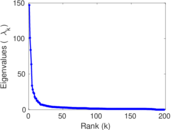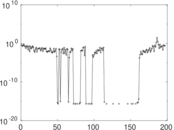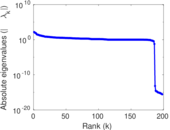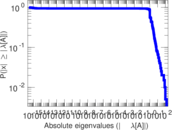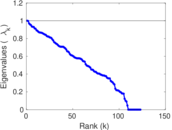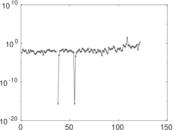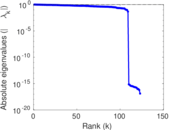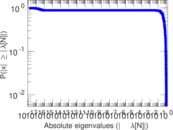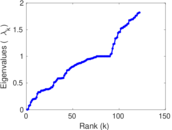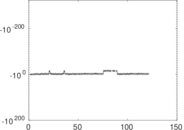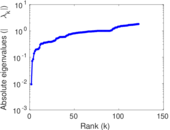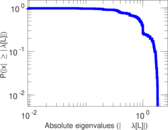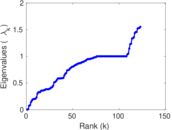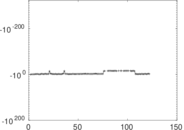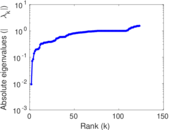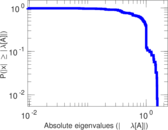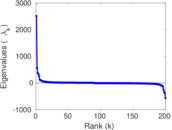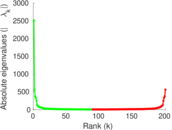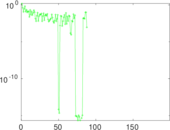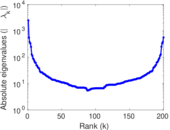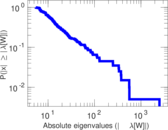# Wikinews edits (sq)

This is the bipartite edit network of the Albanian Wikinews. It contains users and pages from the Albanian Wikinews, connected by edit events. Each edge represents an edit. The dataset includes the timestamp of each edit.

 Code `nsq` Internal name `edit-sqwikinews` Name Wikinews edits (sq) Data source http://dumps.wikimedia.org/ AvailabilityDataset is available for download Consistency checkDataset passed all tests Category Authorship network Dataset timestamp 2017-10-20 Node meaning User, article Edge meaning Edit Network formatBipartite, undirected Edge typeUnweighted, multiple edges Temporal dataEdges are annotated with timestamps

## Statistics

 Size n = 2,511 Left size n1 = 200 Right size n2 = 2,311 Volume m = 7,745 Unique edge count m̿ = 4,986 Wedge count s = 1,808,578 Claw count z = 617,618,660 Cross count x = 169,131,895,143 Square count q = 511,837 4-Tour count T4 = 11,350,268 Maximum degree dmax = 2,152 Maximum left degree d1max = 2,152 Maximum right degree d2max = 128 Average degree d = 6.168 86 Average left degree d1 = 38.725 0 Average right degree d2 = 3.351 36 Fill p = 0.010 787 5 Average edge multiplicity m̃ = 1.553 35 Size of LCC N = 2,279 Diameter δ = 12 50-Percentile effective diameter δ0.5 = 2.335 81 90-Percentile effective diameter δ0.9 = 5.916 07 Median distance δM = 3 Mean distance δm = 3.584 25 Gini coefficient G = 0.707 878 Balanced inequality ratio P = 0.231 698 Left balanced inequality ratio P1 = 0.083 666 9 Right balanced inequality ratio P2 = 0.339 961 Relative edge distribution entropy Her = 0.741 017 Power law exponent γ = 2.539 24 Tail power law exponent γt = 3.521 00 Tail power law exponent with p γ3 = 3.521 00 p-value p = 0.000 00 Left tail power law exponent with p γ3,1 = 1.741 00 Left p-value p1 = 0.214 000 Right tail power law exponent with p γ3,2 = 5.691 00 Right p-value p2 = 0.080 000 0 Degree assortativity ρ = −0.247 888 Degree assortativity p-value pρ = 1.055 45 × 10−70 Spectral norm α = 147.021 Algebraic connectivity a = 0.009 379 87 Spectral separation |λ1[A] / λ2[A]| = 1.456 23 Controllability C = 2,134 Relative controllability Cr = 0.851 556

## Plots

### Fruchterman–Reingold graph drawing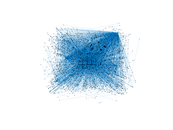### Degree distribution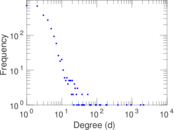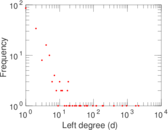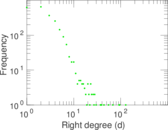### Cumulative degree distribution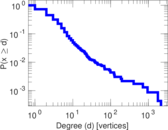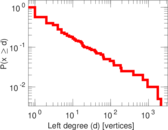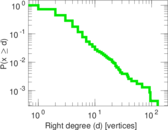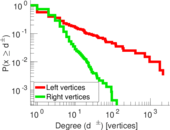### Lorenz curve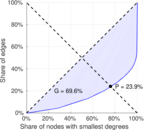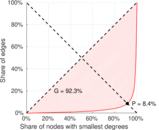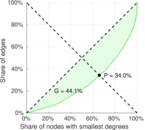### Spectral distribution of the adjacency matrix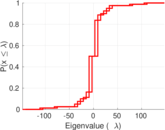### Spectral distribution of the normalized adjacency matrix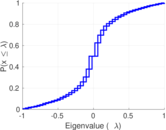### Spectral distribution of the Laplacian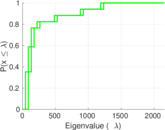### Spectral graph drawing based on the adjacency matrix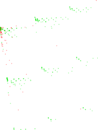### Spectral graph drawing based on the Laplacian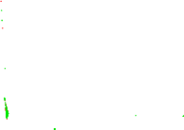### Spectral graph drawing based on the normalized adjacency matrix### Degree assortativity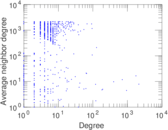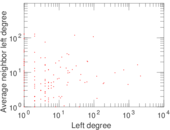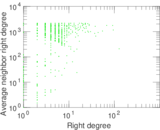### Zipf plot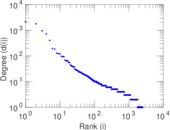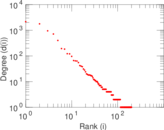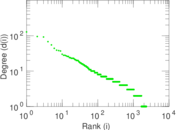### Hop distribution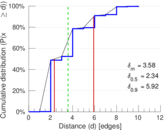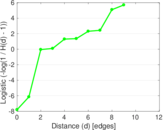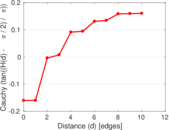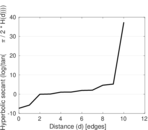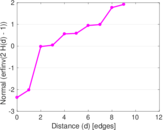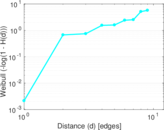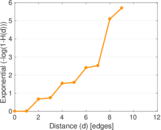### Double Laplacian graph drawing### Delaunay graph drawing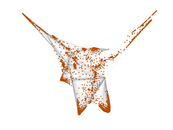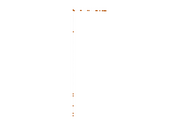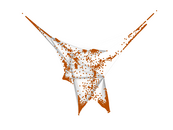### Edge weight/multiplicity distribution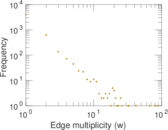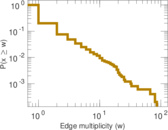### Temporal distribution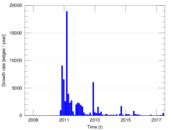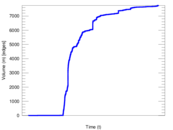### Temporal hop distribution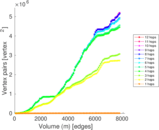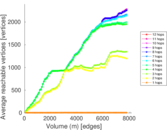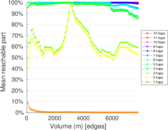### Diameter/density evolution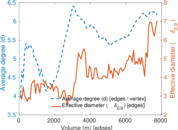### Matrix decompositions plots